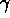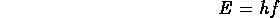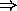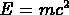PHYSICS 1020 - Spring 1997

SOLUTION TO FINAL EXAM
22 April 1997

1. You are in a spaceship moving past Earth at nearly lightspeed and you observe Mort, who is on Earth. You measure his mass, pulse rate, and size. How have they changed? (Be specific)
As seen from you, Mort is moving past you at nearly light speed. Therefore his mass appears increased by a large factor (the Lorentz factor), his heartbeat has slowed down. His size (in the direction of motion) has shrunk by the same factor, while his size perpendicular to the direction of motion has not changed.

2. Is a circle (meaning the perimeter of a flat circular area) a space?
How many dimensions does it have?
Is it a curved space, or is it flat?
Give an example of a flat one-dimensional space.
A circle is a curved one-dimensional space. A straight line (infinitely long) is a flat one-dimensional space.

3. Explain why ultraviolet light can damage cells in your skin but visible light cannot.
Ultraviolet photons have more energy, enough to disrupt the chemical processes in the cells of your skin. Remember that the energy carried by a photon iswhere f is the frequency and h is Planck's constant. Photons of visible light have a longer wavelengthsmaller frequencysmaller energy than ultraviolet light photons.

4. Suppose that two electrons travel separately through a double-slit apparatus. Your detector finds the first electron at the upper end of the interference pattern, and the other at the lower end. Is it correct to say that the first electron came through the upper slit and the second came through the lower slit? Explain.
It would not be correct to say that one electron came through one slit, while the other went through the other; due to the interference between the electron waves, in some sense both electrons go through both slits. If electrons behaved like particles rather than waves, they would not show interference, and the pattern observed would be the pattern corresponding to the sum of the single slit patterns, i.e. non-interfering.

5. If a very accurate measurement of an atom's mass could be made in an excited state and in its ground state, would you find a difference between the masses? If yes, which one would have a higher mass? Explain. (Hint: remember). What happens to an atom's mass when it emits a photon?
Since an excited atom has more energy than an atom in its ground state, its mass is bigger (because of the mass-energy equivalence). When an atom emits a photon, it loses energy, and therefore its mass decreases.

6. If Planck's constant were smaller than it is, how would this affect the size of atoms? Would atoms be smaller or larger (or stay the same size)? Explain why.
A smaller Planck's constant would allow atoms to be smaller, due to smaller quantum uncertainties. If Planck's constant were zero, there would be no quantum effects - everything would be continuous and smooth, fully predictable in the Newtonian sense, but - we might not exist to be bored by this.

7. In what ways are the W and Z particles similar to photons? In what ways are they different?
Similarities:
They are all exchange particles - ``gauge bosons'' which play a role as mediators of interactions; they all have spin 1.
Differences:
the photon is massless (zero rest mass), while the W and Z are massive; the photon always travels at lightspeed, while the W and Z don't; the photon and Z are neutral, while the W's are charged.

8. In what ways are quarks similar to electrons? In what ways are they different?
Similarities:
Both quarks and electrons are fermions, i.e they have half-integer spin; they both are matter particles, i.e. they are the basic constituents of material objects.
They both are ``massive'', i.e. their rest mass is non-zero (i.e. they move at less than lightspeed).
They both appear to be ``point-like'', i.e. there is no evidence for them to have any substructure (but this may just mean that we haven't yet looked close enough).
Differences:
Quarks have fractional electric charges (-1/3, 2/3), while electrons have integer charge (in terms of proton charges).
Quarks carry color charge, i.e. they feel the strong interaction, while electrons don't.

9. Does a semiconductor become a better conductor as the temperature rises? Justify your answer.